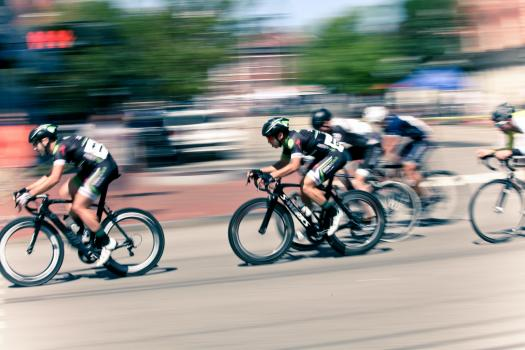# Derivatives And 1d Motion

10 Questions | Attempts: 159SettingsHow well do you do with derivatives and 1d motion? Do you believe you can pass this quiz? With this quiz, you should determine the relationships between acceleration and position and speed and velocity. You will also be expected to understand the nature of velocity with respect to time and solve equations concerning acceleration. This quiz may be challenging, but it is worth a shot.

• 1.
Which statement correctly describes the relationship between position and acceleration?
• A.

Acceleration is the rate of change of position.

• B.

Position is the second derivative of acceleration with respect to time.

• C.

Acceleration is the time derivative of the rate of change of position.

• D.

Position and acceleration are not related. It's velocity which is the derivative of position wirh respect to time.

• 2.
Which of the following can be found starting by taking the derivative of velocity with respect to time? Mark ALL that apply. (No integration allowed...i.e., no claiming it can be found by taking the derivative and then undoing the derivative!)
• A.

Instantaneous acceleration

• B.

Average acceleration

• C.

Average velocity

• D.

Maximum velocity

• E.

Displacement

• F.

Speed

• G.

Intervals of increasing or decreasing velocity

• H.

Intervals when the object is moving forward vs. backward

• 3.
The acceleration function for an object undergoing rectilinear motion is a(t) = 3t2+t – 3. Which of the following functions could represent the velocity function for the object?
• A.

A(t)

• B.

B(t)

• C.

C(t)

• D.

D(t)

• E.

F(t)

• 4.
The position of a particle moving along the x-axis is given by x(t) = t(t – 5)2. What is the particle's average velocity during the time interval [0,4]?
• A.

0

• B.

1

• C.

–3.5

• D.

–7

• 5.
The position of a particle moving along the x-axis is given by x(t) = t(t – 5)2. What is the particle's acceleration at t=2?
• A.

–12

• B.

–8

• C.

–3

• D.

Not enough information

• 6.
A cannonball is launched from the prow of a ship such that its height above water is given by h(t) = 10 + 207t – 4.9t2Which equation could you solve to find (directly) the time when the cannonball reaches its maximum height?
• A.

H(t) = 0

• B.

H'(t)=0

• C.

H''(t)=0

• D.

H(t) = h'(t)

• 7.
A cannonball is launched from the prow of a ship such that its height above water is given by h(t) = 10 + 207t – 4.9t2What is the maximum vertical velocity of the cannonball for its flight through the air?
• A.

~5 m/s

• B.

~10 m/s

• C.

~200 m/s

• D.

~2200 m/s

• 8.
A cannonball is launched from the prow of a ship such that its height above water is given by h(t) = 10 + 207t – 4.9t2What is the maximum vertical speed of the cannonball for its flight through the air?
• A.

–207.5 m/s

• B.

–207 m/s

• C.

207 m/s

• D.

207.5 m/s

• 9.
The graphs show the position, velocity, and acceleration of an object moving on a line.
• A.

Graph A is the position, Graph B is the velocity, Graph C is the acceleration

• B.

Graph C is the position, Graph B is the velocity, Graph A is the acceleration

• C.

Graph B is the position, Graph A is the velocity, Graph C is the acceleration

• D.

Graph A is the position, Graph C is the velocity, Graph B is the accleration

• 10.
Which statement correctly describes the relationship between speed and velocity?
• A.

Speed is the rate of change of velocity.

• B.

Velocity is the rate of change of speed.

• C.

Velocity is the accumulation of speed.

• D.

Speed is the magnitude of velocity.

## Related TopicsBack to top
×

Wait!
Here's an interesting quiz for you.